# Handling US Tax Depreciation in SAP (Part 5): Mid-Period and Mid-Quarter Convention

I’ve covered the basics of how SAP can track and manage asset values to support US tax valuations.  The last blog talked about making prior year adjustments which might be the number one question we have to field from tax professionals.  For this blog I’m going to dive back into the SAP depreciation calculation (always great fun!) regarding some of the niche US tax calculation requirements.

```Blog Series:
Handling US Tax Depreciation in SAP (Part 1): The Basics
Handling US Tax Depreciation in SAP (Part 2): Separate Books
Handling US Tax Depreciation in SAP (Part 3): Basis Adjustments
Handling US Tax Depreciation in SAP (Part 4): Prior Year AdjustmentsHandling US Tax Depreciation in SAP (Part 5): Mid-Period and Mid-Quarter ConventionHandling US Tax Depreciation in SAP (Part 6): IRS Passenger VehiclesHandling US Tax Depreciation in SAP (Part 7): Tax Forms 4562 & 4797```

### In Life (and Taxes), It’s All About the Timing

The IRS makes it clear that when an asset begins and ends calculating depreciation is a key factor in the final tax rate that should be chosen.  The good news is that SAP treats assets in the same way.  When an asset starts depreciating, ends depreciating, or switches over to another depreciation convention are all extremely important variables in the final calculation.

The first blog walked through some basic MACRS and AMT calculations.  Those were based on a mid-year convention so I’ll switch over to mid-period (or mid-month) and mid-quarter just to show that SAP can easily handle these alternative timing requirements too.

### Mid-Month

First, I’ll cover mid-month.  The asset below is a non-residential piece of property depreciating using the MACRS SL mid-month convention.  For demo purposes only I’ve limited the UL to 10 years in order to show the correct first and last year’s worth of partial depreciation.  A 31 or 39 year life asset is just too long of a scrollbar to capture in a screenshot so I’m keeping it easy and just using 10 years.

• APC: \$120,000 USD
• UL:  10 years (120 periods)
• Capitalization Date:  January 1, 2020
• Annual Depreciation:  \$120,000 / 10 years = \$12,000
• Monthly Depreciation:  \$12,000 / 12 periods = \$1,000
• Calculation:  Since this is straight line, the calculation works out to a consistent \$1,000 USD per period.  However for a mid-month calculation, the asset only gets a half of the first month’s calculation which is \$500.  As you can see in the screenshot below, the year 1 depreciation is 11 full months (11 x 1,000) and 1 half month (1/2 x 1,000) which totals to \$11,500.  Years 2 through 10 get a full 12,000 per year with the remaining single half-month in year 11 of \$500.  This is all correct.Just for grins, here is the same asset after I changed the life to 39 years.  I can’t show the final year as I mentioned above but the calculation is correct if you walk through the math below.

• Annual Depreciation = \$120,000 / 39 years = \$3,076.92
• Monthly Depreciation = \$3,076.92 / 12 periods = \$256.41
• Half-Month Depreciation = \$256.41 / 2 half-periods = \$128.21
• Year 1 Depreciation = 11 full periods at \$256.41 + 1 half-period at \$128.21 = \$2,948.72
• Years 2-39 = Full annual depreciation of \$3,076.92
• Year 40 (not shown) = A single half-month of depreciation at \$128.21### What Makes This Hard?

The part that makes this hard in SAP and is routinely messed up during an implementation is the calculation at a level lower than a month.  In this system, and most systems for that matter, the company code is using a 12 period fiscal year variant (FYV).  It doesn’t matter if it’s a ‘normal’ variant that runs Jan-Dec or some alternative variant such as the Feb-Jan schedule common in the retail industry.  Actually, the number of periods doesn’t matter either.  You could easily be running a 13 period FYV if that’s what is required.  The point is that the variant is defined with a certain number of periods (12 in my example) which is therefore the lowest level of detail that the depreciation engine can use to calculate an amount.

Below is a screenshot that shows the FYV I’m using (K4) and it’s defined with 12 fiscal periods.Obviously, if you’re determining the depreciation rate from the useful life of the asset, then the number of periods in the year (the denominator) is a key mathematical variable!  When the depreciation engine is calculating the annual amount (\$12,000 in the first 10 year example) and it then wants to calculate/display it per month (\$1,000/month), it’s going to divide by the number of posting periods (12).

But for the mid-month and mid-quarter calculations, you have to only take half of the depreciation in the first month.  How is that possible?  When you’ve already determined a monthly amount, how do you re-calculate it again?  If a period has been defined as one month, how to calculate something at a lower level?  That’s the hard part.

Hard but not impossible!  There are actually a couple of configuration options to get this right but few folks do it correctly.  What I want to stress to the tax professionals reading this is that you need to create a new asset and use your MACRS SL depreciation key (either SAP delivered or custom) and then double check the first year’s depreciation.  Did it take the entire first month or did it correctly only calculate half?  It’s simple to calculate offline and simple to confirm at the Asset Explorer (AW01N).

Now, back to the demo and the mid-quarter calculation.

### Mid-Quarter

From a calculation perspective, mid-quarter is nearly the same thing as mid-period.  The only difference is that instead of having to split the middle of the capitalization month, it’s the middle of the quarter… but that happens to be the middle of a month, just not the capitalization month.  For SAP, it still has to only grant a half month of depreciation in the first month.

Here’s an example.

• APC:  \$100,000 USD
• UL:  5 years (60 periods)
• Capitalization Date:  February 19, 2021.  Using a normal calendar, this places the capitalization in the first quarter.

Below is the depreciation schedule from the IRS for assets acquired in the first quarter and depreciating using the mid-quarter convention.  I’m referencing the rates in the 2nd column under “5-year”.  Note, this is from the 2019 publication but the rates haven’t ever changed for the mid-quarter calculation because the math principles behind them haven’t changed.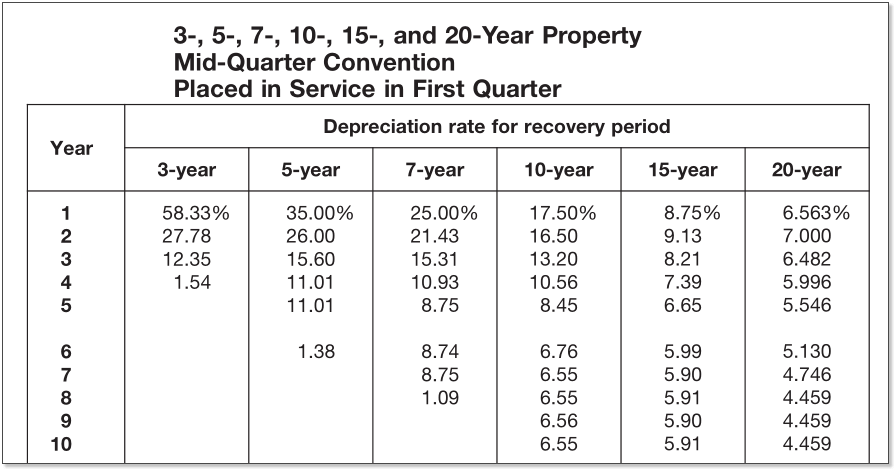And here is the asset in SAP with the same figures.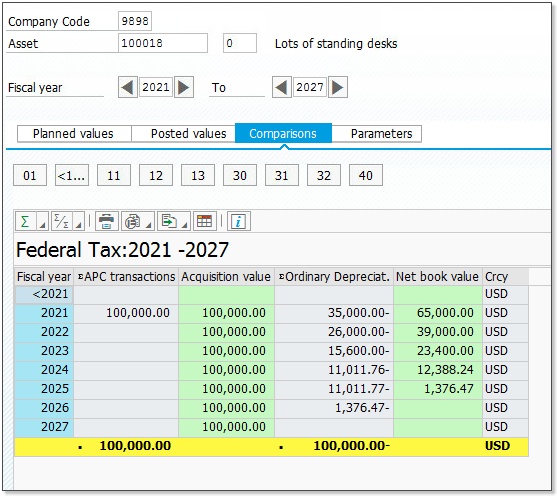Just to follow through on this all the way to the end, here are the rates and an example asset in SAP for the other three quarters.  They all tie out.

#### 2nd Quarter Acquisition: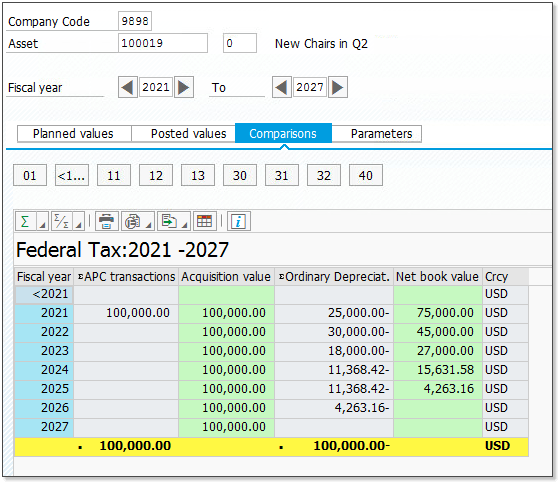#### 3rd Quarter Acquisition: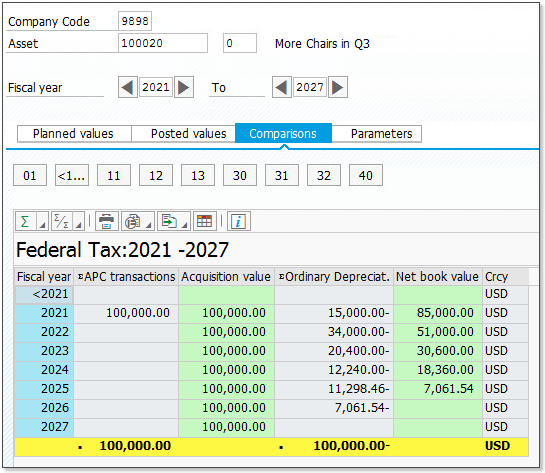#### 4th Quarter Acquisition: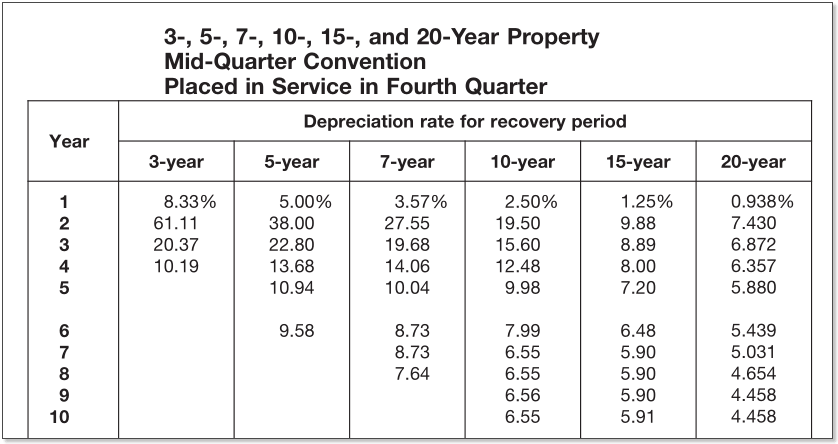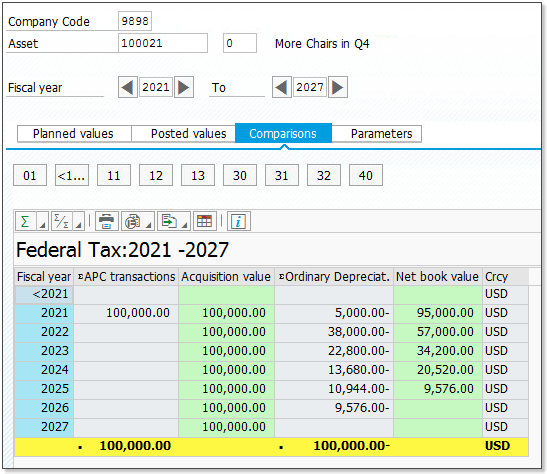### Conclusion

As you can see, the values above all tie out and are even more precise than what the IRS requires.  If you wanted to implement some rounding to tie to the IRS tables, that can be done via configuration and localized on the tax books without impacting the corporate book.

In the end, there is no reason not to keep this information in SAP.  That said, it’s often done incorrectly so you’re going to want to double-check this during testing.  If you have questions on it don’t hesitate to reach out to us.